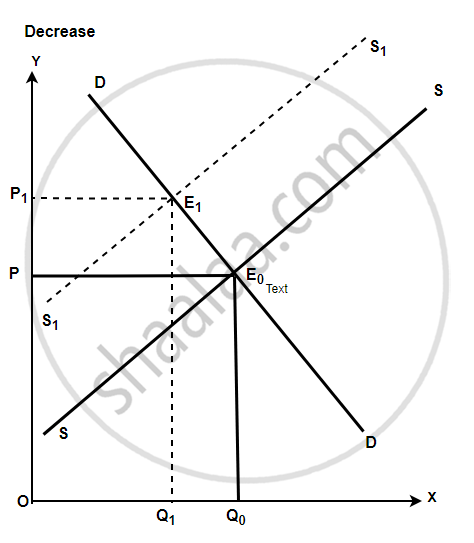# Answer the Following Question. Show with the Help of Diagrams, the Effect on Equilibrium Price and Quantity When: There is a Rise in the Prices of Inputs. - Economics

Short Note

Show with the help of diagrams, the effect on equilibrium price and quantity when:
There is a rise in the prices of inputs.

#### Solution

With a rise in the price of the input, the cost of production will increase and profit will fall. With a fall in profit rate supply will decrease.
A new equilibrium will be established which will be at a higher price, lower quantity demand and lower supply.Concept: Market Equilibrium
Is there an error in this question or solution?
2014-2015 (March) Set 1

Share